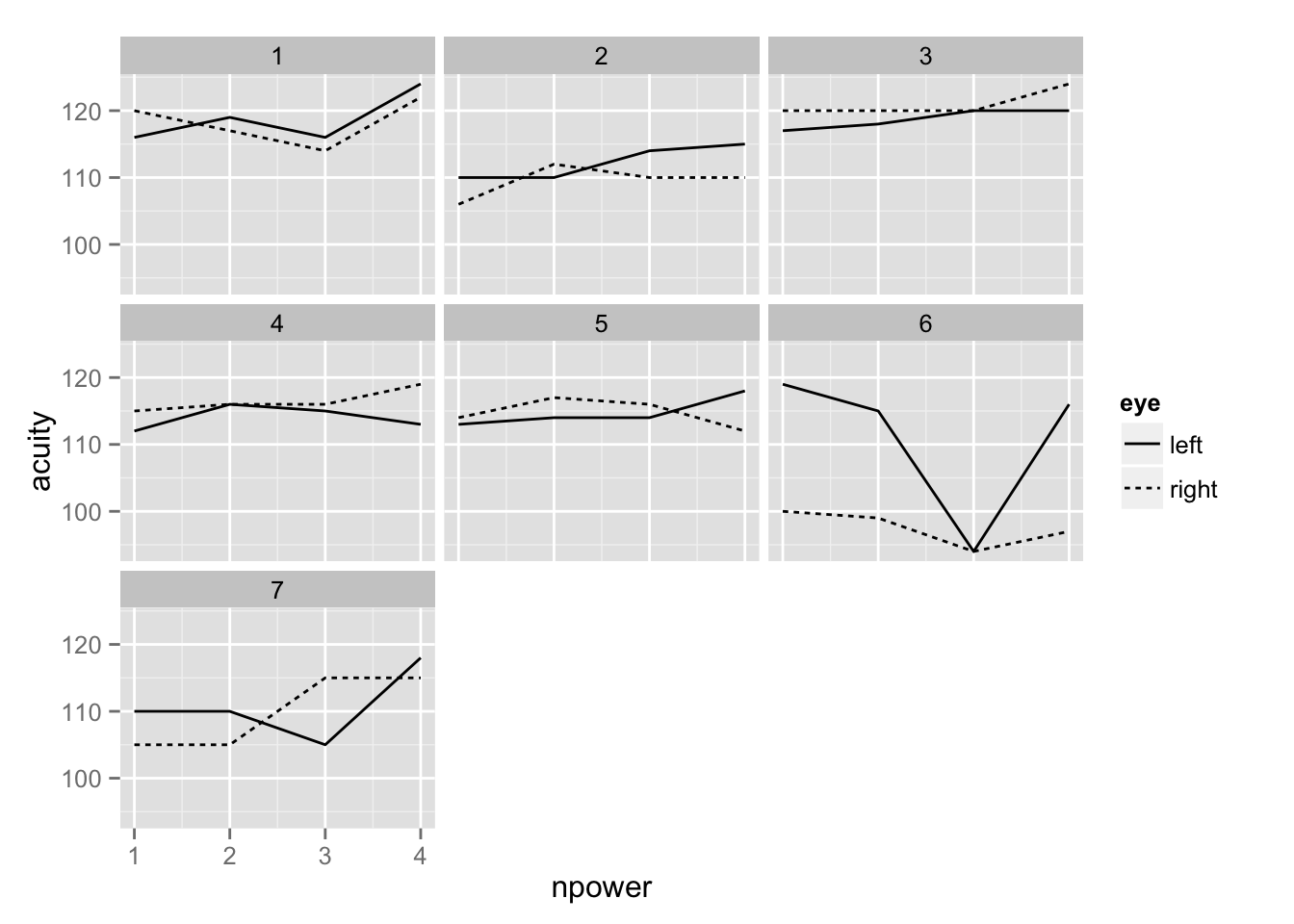`` "Created: Wed Apr  1 16:47:20 2015"``

See the introduction for an overview.

``````library(lme4)
library(ggplot2)
options(digits=5,show.signif.stars=FALSE)``````

Load in and summarize the data:

``````data(vision, package="faraway")
vision\$npower <- rep(1:4,14)
summary(vision)``````
``````     acuity     power       eye     subject     npower
Min.   : 94   6/6 :14   left :28   1:8     Min.   :1.00
1st Qu.:110   6/18:14   right:28   2:8     1st Qu.:1.75
Median :115   6/36:14              3:8     Median :2.50
Mean   :113   6/60:14              4:8     Mean   :2.50
3rd Qu.:118                        5:8     3rd Qu.:3.25
Max.   :124                        6:8     Max.   :4.00
7:8                   ``````

Plot the data:

``ggplot(vision, aes(y=acuity, x=npower, linetype=eye))+geom_line()+facet_wrap(~ subject)``## Fit the model

``````mmod <- lmer(acuity~power+(1|subject)+(1|subject:eye),vision)
print(summary(mmod),corr=FALSE)``````
``````Linear mixed model fit by REML ['lmerMod']
Formula: acuity ~ power + (1 | subject) + (1 | subject:eye)
Data: vision

REML criterion at convergence: 328.7

Scaled residuals:
Min     1Q Median     3Q    Max
-3.424 -0.323  0.011  0.441  2.466

Random effects:
Groups      Name        Variance Std.Dev.
subject:eye (Intercept) 10.3     3.21
subject     (Intercept) 21.5     4.64
Residual                16.6     4.07
Number of obs: 56, groups:  subject:eye, 14; subject, 7

Fixed effects:
Estimate Std. Error t value
(Intercept)  112.643      2.235    50.4
power6/18      0.786      1.540     0.5
power6/36     -1.000      1.540    -0.6
power6/60      3.286      1.540     2.1``````
``anova(mmod)``
``````Analysis of Variance Table
Df Sum Sq Mean Sq F value
power  3    141    46.9    2.83``````

Estimates but no inferential output as expected.

Parametric bootstrap-based confidence intervals:

``confint(mmod, method="boot")``
``````                               2.5 %   97.5 %
sd_(Intercept)|subject:eye   0.00000   5.3654
sd_(Intercept)|subject       0.00000   8.0743
sigma                        3.16913   4.9847
(Intercept)                108.27293 117.2798
power6/18                   -2.11527   3.9079
power6/36                   -3.98635   2.1837
power6/60                    0.31575   6.5202``````

## pbkrtest package

We execute the KR adjusted test in this example:

``````library(pbkrtest)
mmod <- lmer(acuity~power+(1|subject)+(1|subject:eye),vision,REML=FALSE)
nmod <- lmer(acuity~1+(1|subject)+(1|subject:eye),vision,REML=FALSE)
KRmodcomp(mmod, nmod)``````
``````F-test with Kenward-Roger approximation; computing time: 0.16 sec.
large : acuity ~ power + (1 | subject) + (1 | subject:eye)
small : acuity ~ 1 + (1 | subject) + (1 | subject:eye)
stat   ndf   ddf F.scaling p.value
Ftest  2.83  3.00 39.00         1   0.051``````

The p-value exceeding 5% is interesting since it contrasts with the previous results being just below 5%. In truth, the result is borderline either way but of course, which side of the fence you are on will matter greatly to some. We might also try the parametric bootstrap.

## Testing the random effects

`RLRsim` cannot be used because there is more than one random effect.

We can implement the parametric bootstrap directly to test whether there is any variance in the eye within subject random effect term.

First we compute the observed LRT statistic

``````mmod <- lmer(acuity~power+(1|subject)+(1|subject:eye),vision,REML=FALSE)
rmod <- lmer(acuity~power+(1|subject),vision,REML=FALSE)
(olrt <- 2*(logLik(mmod)-logLik(rmod)))``````
``'log Lik.' 6.9608 (df=7)``

Now we resample 1000 times and computed the p-value:

``````lrstat <- numeric(1000)
for(i in 1:1000){
racuity <- unlist(simulate(rmod))
bmod <-    refit(mmod, racuity)
smod <-    refit(rmod, racuity)
lrstat[i] <- 2*(logLik(bmod)-logLik(smod))
}
mean(lrstat > olrt)``````
`` 0.003``

Looks like there is some variation in the eyes within subjects

## lmerTest package

``````library(lmerTest)
mmod <- lmer(acuity~power+(1|subject)+(1|subject:eye),vision)
print(summary(mmod),corr=FALSE)``````
``````Linear mixed model fit by REML t-tests use Satterthwaite approximations
to degrees of freedom [merModLmerTest]
Formula: acuity ~ power + (1 | subject) + (1 | subject:eye)
Data: vision

REML criterion at convergence: 328.7

Scaled residuals:
Min     1Q Median     3Q    Max
-3.424 -0.323  0.011  0.441  2.466

Random effects:
Groups      Name        Variance Std.Dev.
subject:eye (Intercept) 10.3     3.21
subject     (Intercept) 21.5     4.64
Residual                16.6     4.07
Number of obs: 56, groups:  subject:eye, 14; subject, 7

Fixed effects:
Estimate Std. Error      df t value Pr(>|t|)
(Intercept)  112.643      2.235   8.800   50.40  3.7e-12
power6/18      0.786      1.540  39.000    0.51    0.613
power6/36     -1.000      1.540  39.000   -0.65    0.520
power6/60      3.286      1.540  39.000    2.13    0.039``````
``anova(mmod)``
``````Analysis of Variance Table of type 3  with  Satterthwaite
approximation for degrees of freedom
Sum Sq Mean Sq NumDF DenDF F.value Pr(>F)
power    141    46.9     3    39    2.83  0.051``````

Can test the random effects:

``rand(mmod)``
``````Analysis of Random effects Table:
Chi.sq Chi.DF p.value
subject       2.96      1    0.09
subject:eye   5.98      1    0.01``````

but we prefer the parametric bootstrap result because of problems with asymptotic distribution of the LRT in such cases.

Pairwise differences may be interesting

``difflsmeans(mmod)``
``````Differences of LSMEANS:
Estimate Standard Error   DF t-value Lower CI Upper CI
power 6/6 - 6/18      -0.8          1.540 39.0   -0.51    -3.90    2.329
power 6/6 - 6/36       1.0          1.540 39.0    0.65    -2.12    4.115
power 6/6 - 6/60      -3.3          1.540 39.0   -2.13    -6.40   -0.171
power 6/18 - 6/36      1.8          1.540 39.0    1.16    -1.33    4.901
power 6/18 - 6/60     -2.5          1.540 39.0   -1.62    -5.62    0.615
power 6/36 - 6/60     -4.3          1.540 39.0   -2.78    -7.40   -1.171
p-value
power 6/6 - 6/18    0.613
power 6/6 - 6/36    0.520
power 6/6 - 6/60    0.039
power 6/18 - 6/36   0.253
power 6/18 - 6/60   0.113
power 6/36 - 6/60   0.008``````

## MCMCglmm

The syntax of MCMCglmm requires that nested factors be uniquely labeled to distinguish, in this case, between the left eye of subject one and subject two (as these are different eyes!). We use the expanded prior for the reasons established in our one random effect example.

``````library(MCMCglmm)
vision\$eyesub <- paste0(vision\$eye,vision\$subject)
eprior <- list(R=list(V=1,nu=0.02),G=list(G1=list(V=1,nu=0.02,alpha.V=1000),G2=list(V=1,nu=0.02,alpha.V=1000)))
bmod <- MCMCglmm(acuity ~ power,random=~subject+eyesub,data=vision,verbose=FALSE,prior=eprior,pr=TRUE)
xyplot(log(bmod\$VCV))``````